# (20 points) A) A causal Continuous-time system has the following pole-zero diagram: jw S-plane Re -1...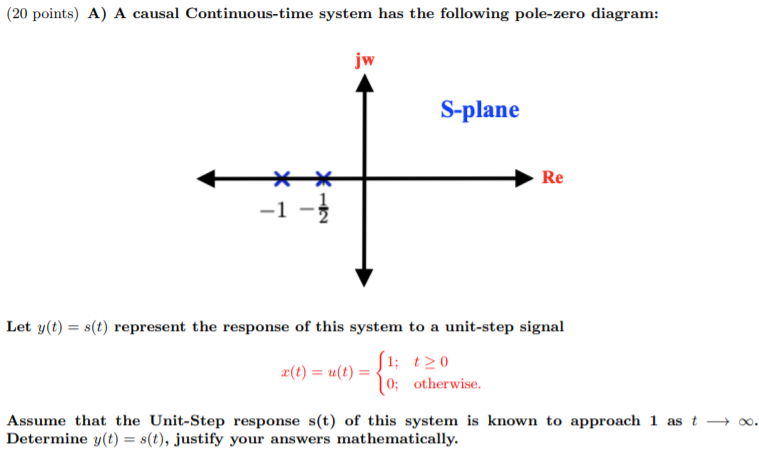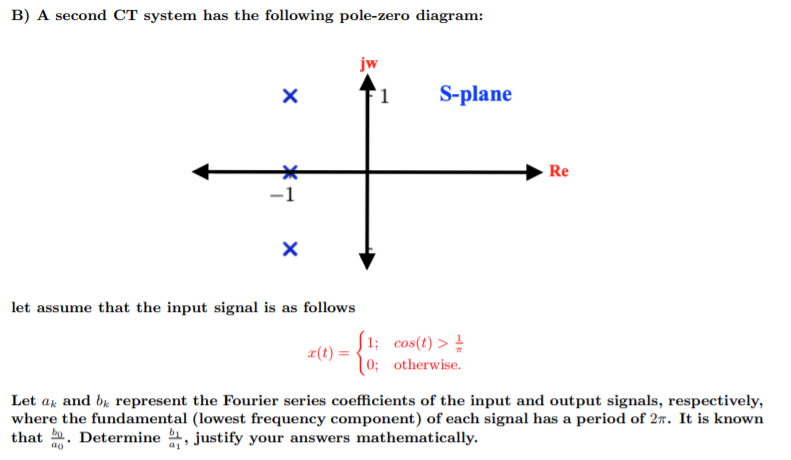(20 points) A) A causal Continuous-time system has the following pole-zero diagram: jw S-plane Re -1 - 2 Let y(t) = s(t) represent the response of this system to a unit-step signal (1; 120 2(t) = u(t) = 0; otherwise. Assume that the Unit-Step response s(t) of this system is known to approach 1 as t + 0. Determine y(t) = s(t), justify your answers mathematically.
B) A second CT system has the following pole-zero diagram: jw x S-plane Re x = { let assume that the input signal is as follows 1; cos(t) > X(t) = 0; otherwise. Let ax and bk represent the Fourier series coefficients of the input and output signals, respectively, where the fundamental (lowest frequency component) of each signal has a period of 2n. It is known that .. Determine , justify your answers mathematically.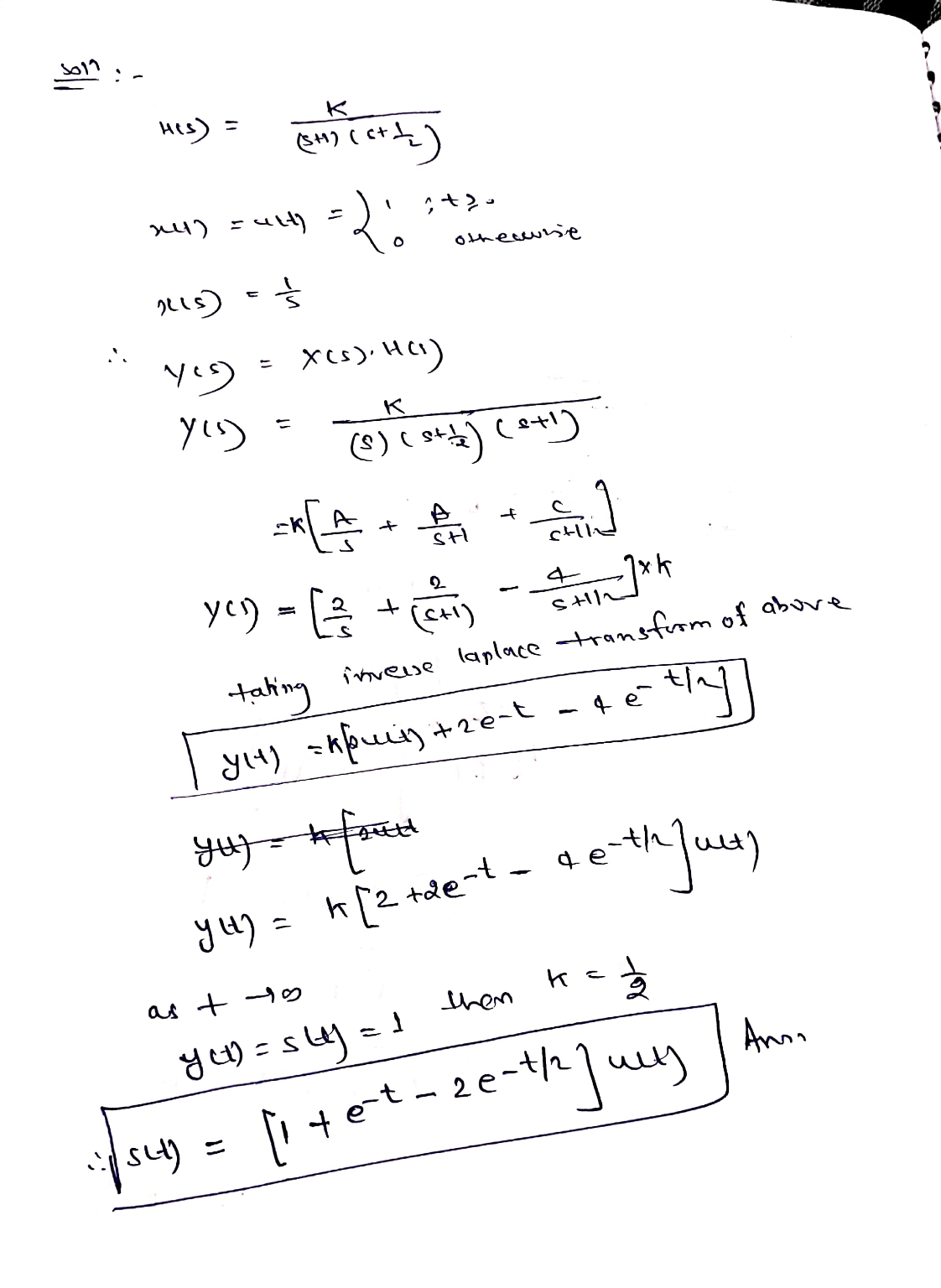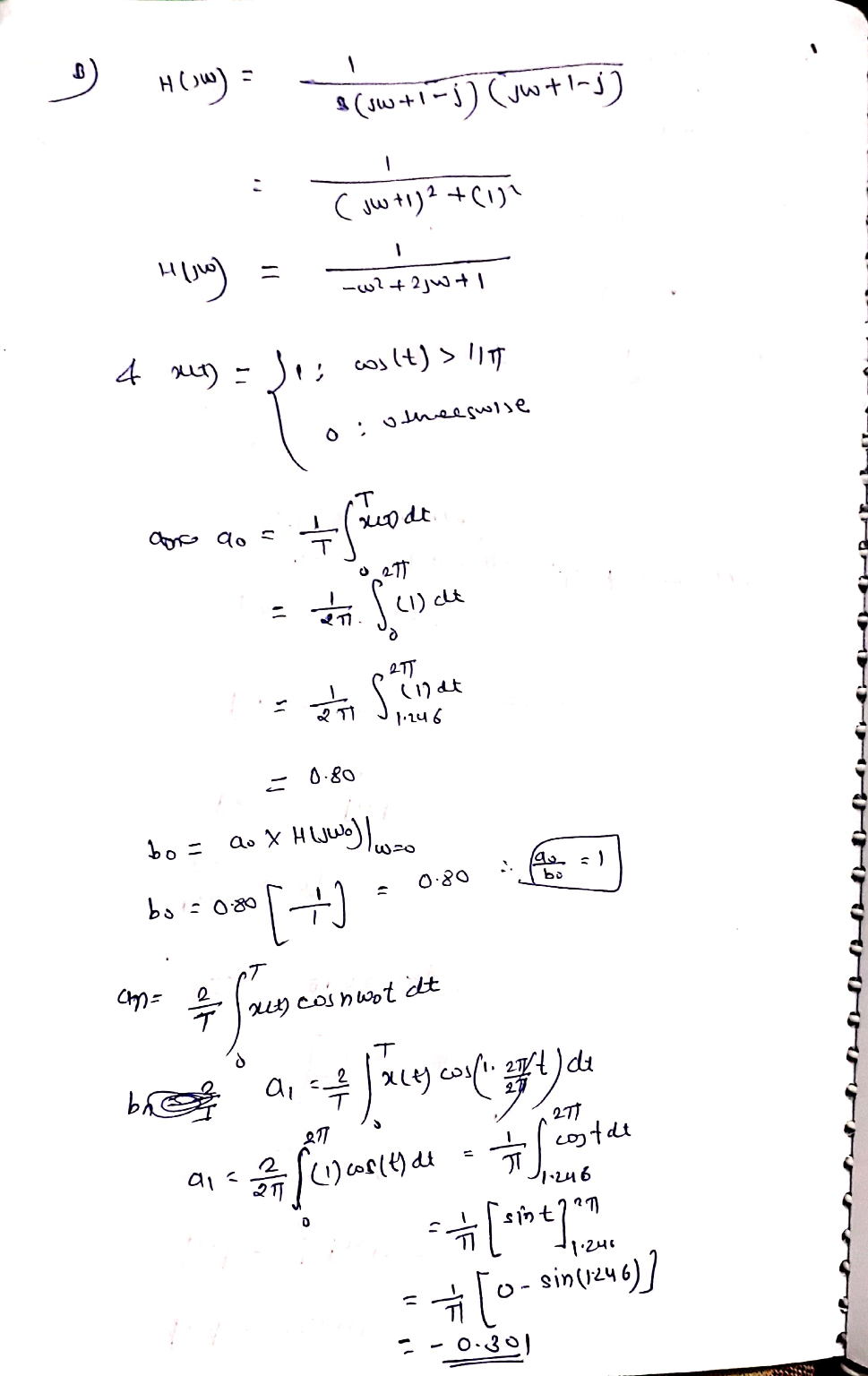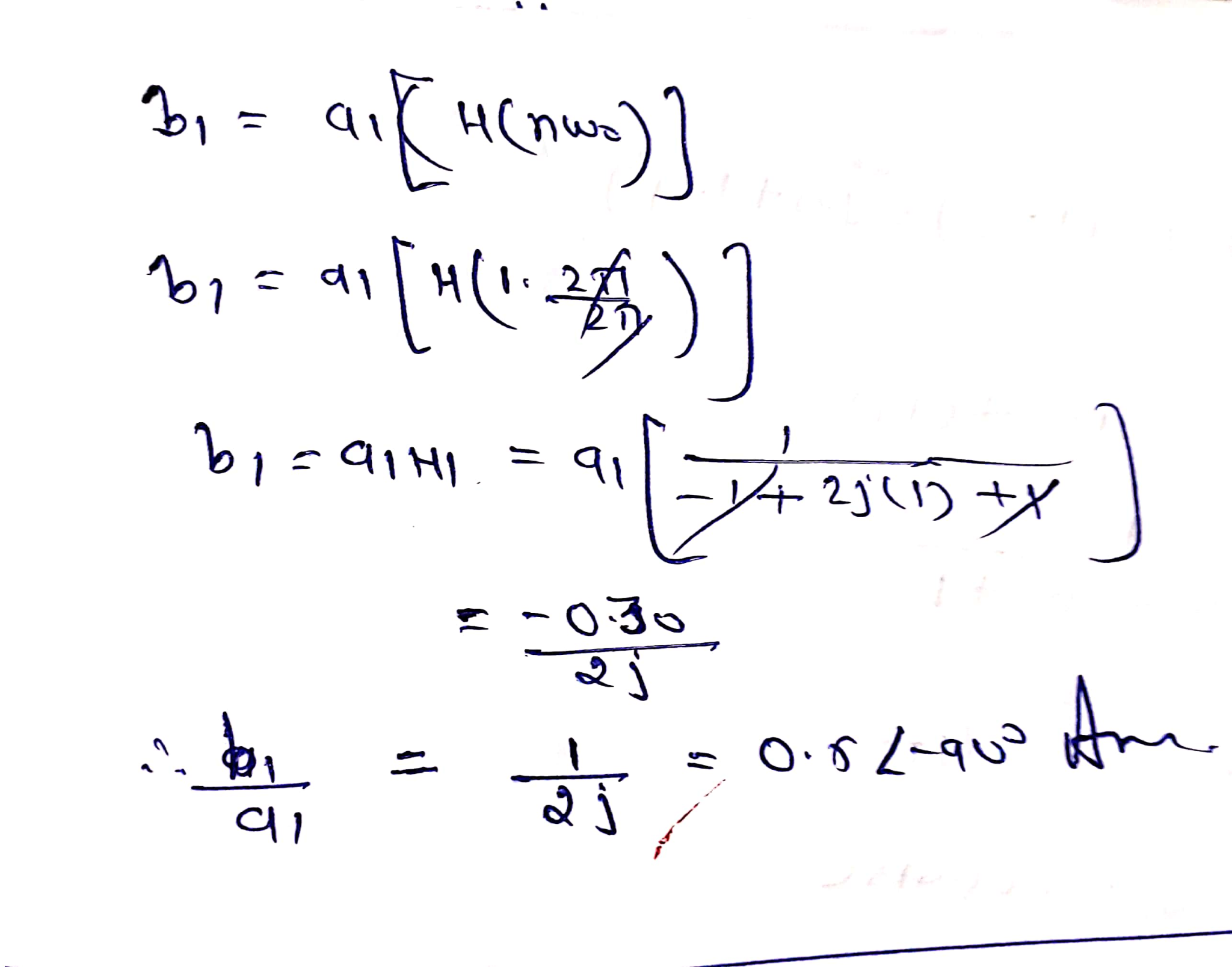##### Add Answer of: (20 points) A) A causal Continuous-time system has the following pole-zero diagram: jw S-plane Re -1...
Similar Homework Help Questions
• ### 5 . A) A causal Continuous-time system has the following pole-zero diagram: jw S-plane Re -1...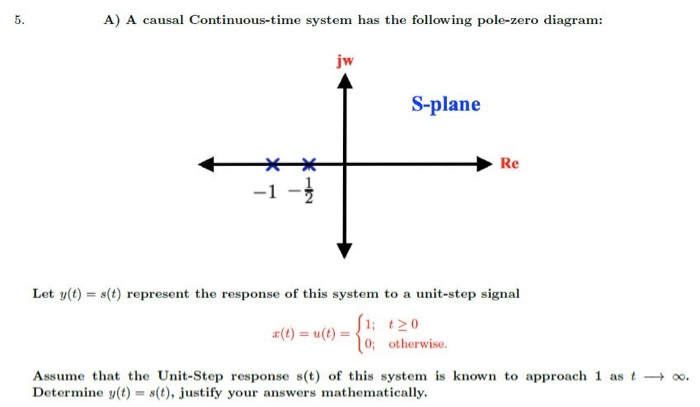5 . A) A causal Continuous-time system has the following pole-zero diagram: jw S-plane Re -1 - Let y(t) = s(t) represent the response of this system to a unit-step signal 0; otherwise. Assume that the Unit-Step response s(t) of this system is known to approach 1 as t o. Determine y(t) = s(t), justify your answers mathematically.

• ### 5. (20 points) A) A causal Continuous-time system has the following pole-zero diagram: jw S-plane Re...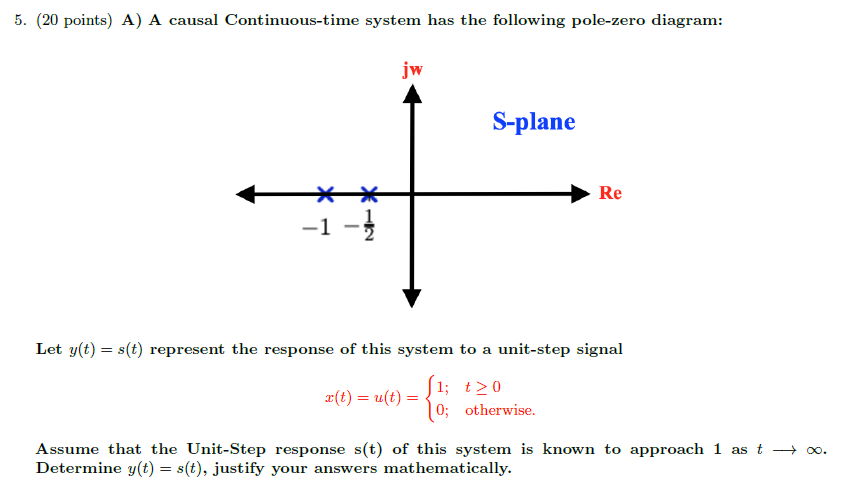5. (20 points) A) A causal Continuous-time system has the following pole-zero diagram: jw S-plane Re * -1 - Let y(t) = s(t) represent the response of this system to a unit-step signal (1; t> 0 *(t) = u(t) = 0; otherwise. Assume that the Unit-Step response s(t) of this system is known to approach 1 as t +. Determine y(t) = s(t), justify your answers mathematically.

• ### Please write very clear 5. (20 points) A) A causal Continuous-time system has the following pole-zero...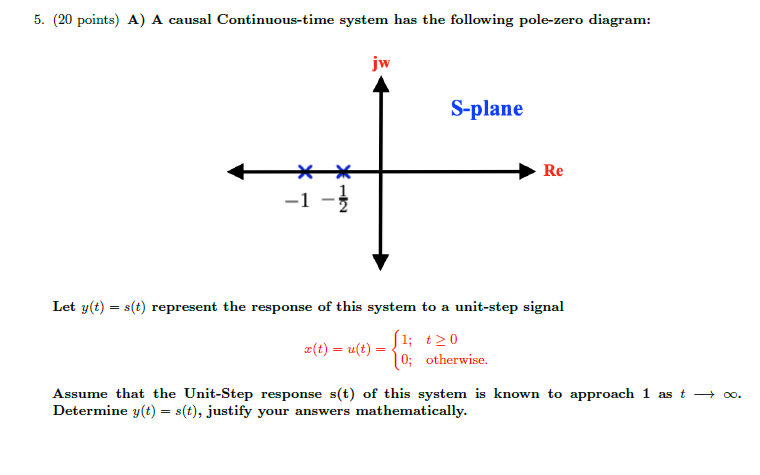Please write very clear 5. (20 points) A) A causal Continuous-time system has the following pole-zero diagram: jw S-plane Re -1 - 1 Let y(t) = s(t) represent the response of this system to a unit-step signal 2(t) = u(t) = 1; to 0; otherwise. Assume that the Unit-Step response s(t) of this system is known to approach 1 as t o. Determine y(t) = s(t), justify your answers mathematically.

• ### B A second CT system has the following pole-zero diagram: jw X S-plane Re х let...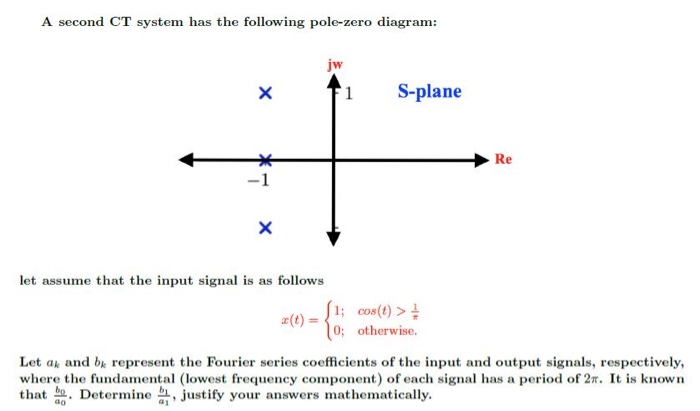B A second CT system has the following pole-zero diagram: jw X S-plane Re х let assume that the input signal is as follows {1; cos(t) > 0; otherwise. Let ak and by represent the Fourier series coefficients of the input and output signals, respectively, where the fundamental (lowest frequency component) of each signal has a period of 27. It is known that he . Determine , justify your answers mathematically.

• ### Q6 A second CT system has the following pole-zero diagram: jw X S-plane Re х let...Q6 A second CT system has the following pole-zero diagram: jw X S-plane Re х let assume that the input signal is as follows {1; cos(t) > 0; otherwise. Let ak and by represent the Fourier series coefficients of the input and output signals, respectively, where the fundamental (lowest frequency component) of each signal has a period of 27. It is known that he . Determine , justify your answers mathematically.

• ### Please write very clear B) A second CT system has the following pole-zero diagram: jw x...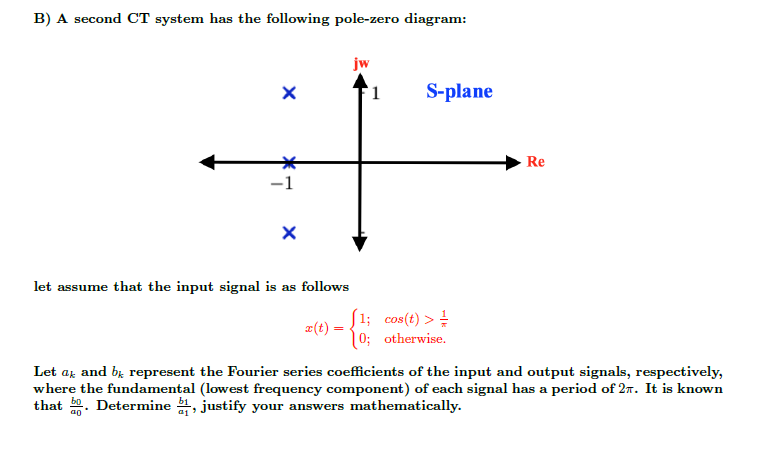Please write very clear B) A second CT system has the following pole-zero diagram: jw x 1 S-plane Re X let assume that the input signal is as follows (1; cos(t) > z(t) 0; otherwise. Let at and be represent the Fourier series coefficients of the input and output signals, respectively, where the fundamental (lowest frequency component) of each signal has a period of 2n. It is known that bo. Determine bo, justify your answers mathematically.

• ### P5.6-3 displays the pole-zero plot of a system that has re 5.6-5 Figure second-order real, causal...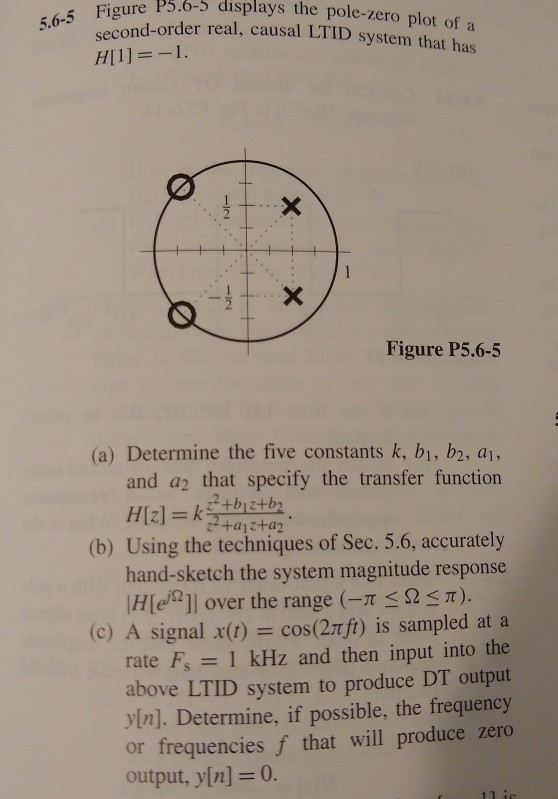P5.6-3 displays the pole-zero plot of a system that has re 5.6-5 Figure second-order real, causal LTID s Figure P5.6-5 (a) Determine the five constants k, bi, b2, aj, and a2 that specify the transfer function (b) Using the techniques of Sec. 5.6, accurately hand-sketch the system magnitude response lH[eill over the range (-π π) (c) A signal x(t) = cos(2πft) is sampled at a rate Fs 1 kHz and then input into the above LTID system to produce DT...

• ### 2.6.1 Consider a causal continuous-time LTI system described by the differential equation u"(t) +...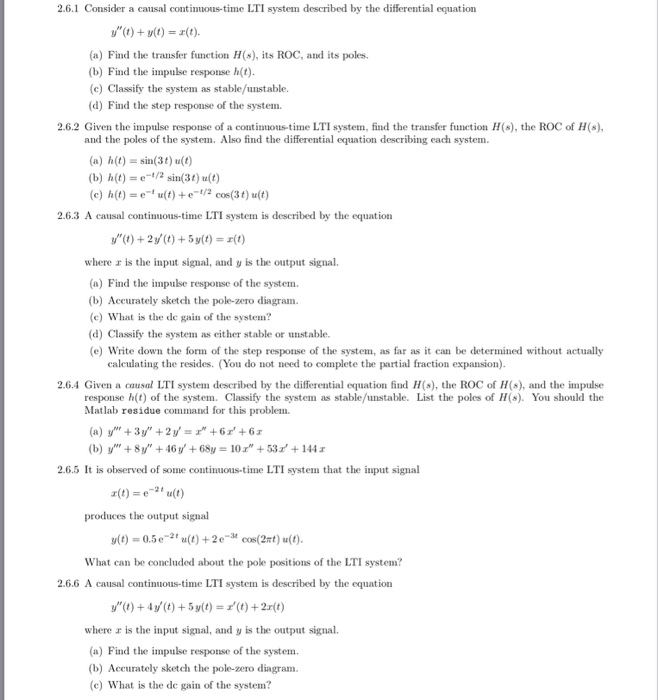2.6.1-2.6.6 2.6.1 Consider a causal continuous-time LTI system described by the differential equation u"(t) + y(t) =エ(t). (a) Find the transfer function H(s), its ROC, and its poles (b) Find the impulse response h(t) (c) Classify the system as stable/unstable. (d) Find the step response of the system. 2.6.2 Given the impulse response of a continuous-time LTI system, find the transfer function H(s), the ROC of H(s) and the poles of the system. Also find the differential equation describing each...

• ### A) Given: H(S) = 32 - 48 +3 52 +8s +12 1. Construct the pole-zero diagram...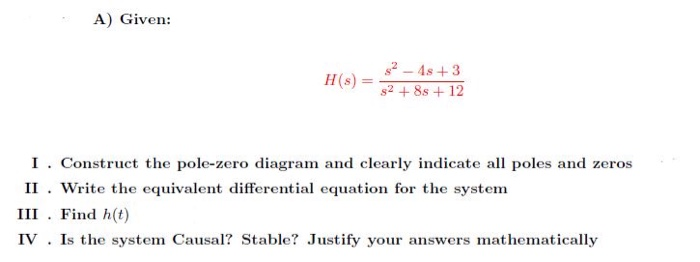A) Given: H(S) = 32 - 48 +3 52 +8s +12 1. Construct the pole-zero diagram and clearly indicate all poles and zeros II . Write the equivalent differential equation for the system III. Find h(t) IV . Is the system Causal? Stable? Justify your answers mathematically

• ### 1.9.10 A causal discrete-time LTI system is described by the equation 1 1 1 vn)(n) I(n-...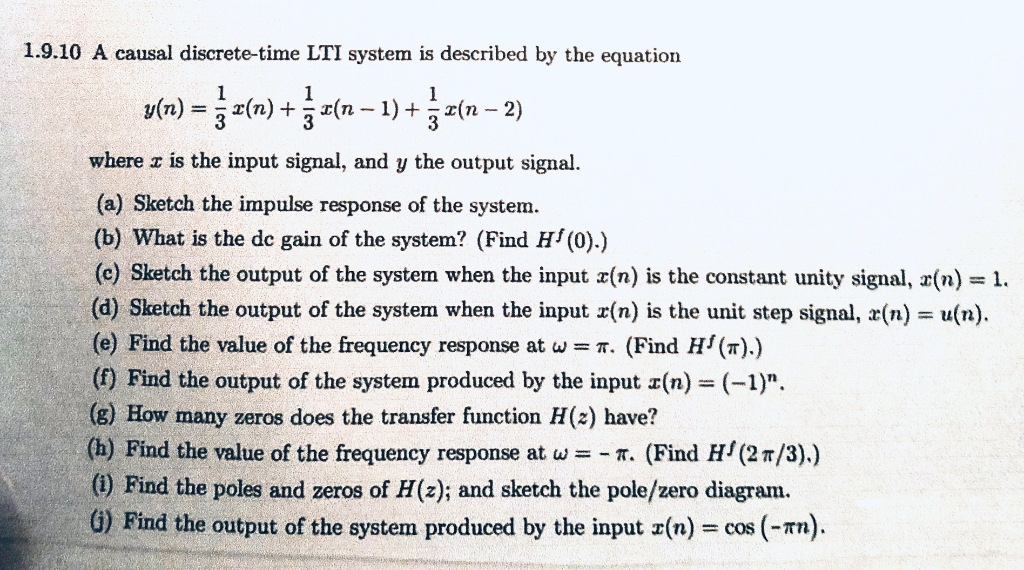1.9.10 A causal discrete-time LTI system is described by the equation 1 1 1 vn)(n) I(n- 1)+ 3 2(n 2) 3 3 where z is the input signal, and y the output signal (a) Sketch the impulse response of the system. (b) What is the de gain of the system? (Find H (0).) (c) Sketch the output of the system when the input x(n) is the constant unity signal, r(n) = 1. (d) Sketch the output of the system when...

Free Homework App